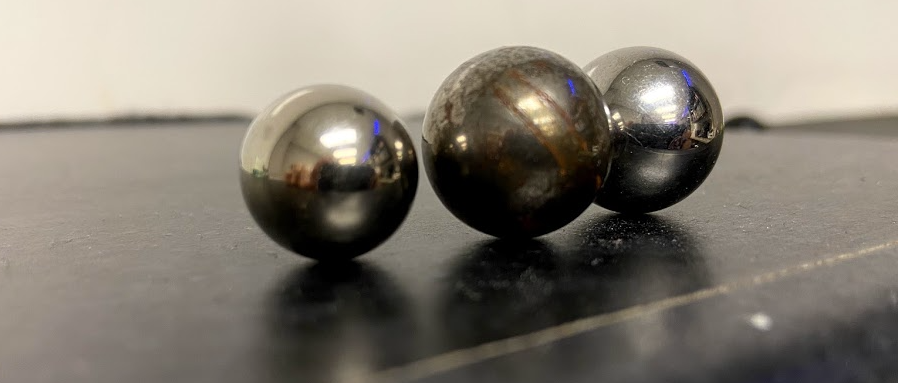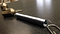# Three Strange Methods to Calculate PiPhoto: Rhett Allain

In the USA, Pi Day is March 14. Why? Because that’s 3/14 in our odd date format system and the first three digits of Pi are 3.14…Note: I’m going to use Pi instead of π because it’s easier to type.

Enough about that. Let’s calculate some Pi. I’m going to share some of my favorite methods to get a value of this awesome number. OK, I’m actually going to start with a fairly straightforward way to measure Pi — just for comparison with the other methods and so that I can give a definition of Pi.

What is Pi?

If you take a circle, there are at least two things you could measure. There is the distance around the outside of the circle (the circumference) and there is the distance across the circle through the middle (the diameter).

With a bigger diameter circle, you get a bigger circumference. In fact, these two variables are proportional for ALL circles. The proportionality constant is Pi.

So, of course you could grab a bunch of circles and measure circumference and diameter — boom, there’s your Pi. But now for some other ways to find Pi.

Pi With Random Numbers.

This is called a Monte Carlo calculation of Pi. The name “Monte Carlo” implies gambling. This method isn’t a gamble, but it does rely on random numbers. Here’s how it works.

Suppose you create two random numbers, both between 0 and 1. Now let these random numbers be an (x,y) coordinate on a plot. You can then calculate the distance from this point to the origin (I will call this r) using the Pythagorean theorem:

Since both x and y can be between 0 and 1, these points will be somewhere is a box that is 1 x 1. However, some of these points will have an r value of less than 1 and some will be greater than 1. This picture should help.

The blue point has an r of greater than 1 and the red is less than 1. The box has an area of 1 (left off the units because it doesn’t matter. But the red points are inside of a quarter circle of radius 1.

Now imagine a whole bunch of points in the box. If they are evenly distributed, then the ratio of points with r less than 1 to the total points should be the same ratio as the area of a quarter circle to the area of a box. Recall that the area of a circle is πr².

I will call the number of points with r less than 1 n-red and I will call the total number of points N. That means we have the following relationship.

That’s it. I just need to make a bunch of random points and calculate their distances to the origin. You could do this by dropping objects on the floor — but that’s super tedious. Instead, I will make a python program. Here is how to do that.

Here’s what it looks like and here is the code.

Although this gives an estimation of the value of Pi, it can take quite a while to get to a decent value. Here is a plot of the calculated value of Pi as a function of the number of points.

It’s not perfect, but it’s not too bad either. Oh, this is a semi-log plot in case you can’t tell.

Finding Pi with a Mass and a Spring

I’m a huge fan of a mass oscillating on a spring. I’ll be honest, just about every physicist loves springs. It’s true.Photo: Rhett Allain. Mass and Spring

The thing about springs (at least ideal springs) is that the force from a spring is proportional to its stretch. We call the proportionality constant, the spring constant (represented by the variable k). That means we can use the following equation for the force.

So, the first step is to find this spring constant. If I hang different masses on the spring and let it settle, then the spring force would be equal to the weight of the mass. From this, I get the following plot.

The slope of this line is the spring constant. Now, I can let the mass oscillate and measure the period (the time for one complete oscillation).

If you work through the math on this problem, it’s possible to show that the period of oscillation (T) depends on both the value of the spring constant (k) and the mass (m) with the following relationship.

Oh, check it out. There’s a Pi in there. If I measure the period, the mass, and the spring constant — I can get Pi. No circles needed. Here is my full explanation with data.

Finding Pi with a Fake Circle

What if you don’t want to measure a real circle? It might be easier to use a computer to create a circle and then measure both the circumference and the radius. From that, you could calculate Pi.

I think if I used some built-in computer function to draw the circle, that would be cheating. I need to make a circle without using circle stuff. OK, here is my plan.

• Model a mass interacting in 2D with a spring.
• The spring is connected to some fixed location and this makes the mass move around in a circle — an orbit.

But how do you find the initial mass velocity so that it makes a circular orbit and not some other type of motion? Instead of calculating the proper initial conditions, I’m going to let it find it’s own circle. I’m just going to add a radial drag force. This is some type of force that only depends on the velocity towards or away from the center of the circle. It’s like radial friction.

Here, check it out.

It seems to work. This is what it looks like when it is “finding” a circular orbit.

Once I have a circle, then I can measure the radius of the circle as the average distance from the center. The circumference is just a some of the step distances during the circle.

It mostly works. From this, I get a Pi value of 3.13791 — it’s not terrible. I think my biggest problem is finding exactly when the mass has made a complete circle. But I will leave that as a homework for you.

Bonus: The Euler Identity

This isn’t a way to find Pi, but it shows Pi in one of the most awesome equations.

I mean, what? This is the Euler Identity. It includes the five coolest numbers. “e” is the natural number such that the slope of the function f(x)=e^x is also e^x. “i” is the imaginary number where i = sqrt(-1). 1 and 0 are of course the numbers one and zero.

It’s just crazy that these numbers are connected by a single equation.

Physics faculty, science blogger of all things geek. Technical Consultant for CBS MacGyver and MythBusters. Former WIRED blogger.

## More from Rhett Allain

Physics faculty, science blogger of all things geek. Technical Consultant for CBS MacGyver and MythBusters. Former WIRED blogger.